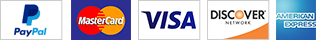ELECT ERIC R. COLEMAN ELECT ERIC R. COLEMAN ELECT ERIC R. COLEMAN ELECT ERIC R. COLEMAN ELECT ERIC R. COLEMAN ELECT ERIC R. COLEMAN ELECT ERIC R. COLEMAN ELECT ERIC R. COLEMAN ELECT ERIC R. COLEMAN ELECT ERIC R. COLEMAN ELECT ERIC R. COLEMAN ELECT ERIC R. COLEMAN ELECT ERIC R. COLEMAN ELECT ERIC R. COLEMAN ELECT ERIC R. COLEMAN ELECT ERIC R. COLEMAN ELECT ERIC R. COLEMAN ELECT ERIC R. COLEMAN ELECT ERIC R. COLEMAN ELECT ERIC R. COLEMAN ELECT ERIC R. COLEMAN ELECT ERIC R. COLEMAN ELECT ERIC R. COLEMAN ELECT ERIC R. COLEMAN ELECT ERIC R. COLEMAN ELECT ERIC R. COLEMAN ELECT ERIC R. COLEMAN ELECT ERIC R. COLEMAN ELECT ERIC R. COLEMAN ELECT ERIC R. COLEMAN ELECT ERIC R. COLEMAN ELECT ERIC R. COLEMAN ELECT ERIC R. COLEMAN ELECT ERIC R. COLEMAN ELECT ERIC R. COLEMAN ELECT ERIC R. COLEMAN ELECT ERIC R. COLEMAN ELECT ERIC R. COLEMAN ELECT ERIC R. COLEMAN ELECT ERIC R. COLEMAN ELECT ERIC R. COLEMAN ELECT ERIC R. COLEMAN ELECT ERIC R. COLEMAN ELECT ERIC R. COLEMAN ELECT ERIC R. COLEMAN ELECT ERIC R. COLEMAN ELECT ERIC R. COLEMAN ELECT ERIC R. COLEMAN ELECT ERIC R. COLEMAN ELECT ERIC R. COLEMAN ELECT ERIC R. COLEMAN ELECT ERIC R. COLEMAN ELECT ERIC R. COLEMAN ELECT ERIC R. COLEMAN ELECT ERIC R. COLEMAN ELECT ERIC R. COLEMAN ELECT ERIC R. COLEMAN ELECT ERIC R. COLEMAN ELECT ERIC R. COLEMAN ELECT ERIC R. COLEMAN ELECT ERIC R. COLEMAN ELECT ERIC R. COLEMAN ELECT ERIC R. COLEMAN ELECT ERIC R. COLEMAN ELECT ERIC R. COLEMAN ELECT ERIC R. COLEMAN ELECT ERIC R. COLEMAN ELECT ERIC R. COLEMAN ELECT ERIC R. COLEMAN ELECT ERIC R. COLEMAN ELECT ERIC R. COLEMAN ELECT ERIC R. COLEMAN ELECT ERIC R. COLEMAN

# Vote for Eric R. Coleman for Senate District 32!

From Collierville to Covington, vote for the Democratic Party!  It is time for more diverse and inclusive representation!

ELECT ERIC R. COLEMAN ELECT ERIC R. COLEMAN ELECT ERIC R. COLEMAN ELECT ERIC R. COLEMAN ELECT ERIC R. COLEMAN ELECT ERIC R. COLEMAN ELECT ERIC R. COLEMAN ELECT ERIC R. COLEMAN ELECT ERIC R. COLEMAN ELECT ERIC R. COLEMAN ELECT ERIC R. COLEMAN ELECT ERIC R. COLEMAN ELECT ERIC R. COLEMAN ELECT ERIC R. COLEMAN ELECT ERIC R. COLEMAN ELECT ERIC R. COLEMAN ELECT ERIC R. COLEMAN ELECT ERIC R. COLEMAN ELECT ERIC R. COLEMAN ELECT ERIC R. COLEMAN ELECT ERIC R. COLEMAN ELECT ERIC R. COLEMAN ELECT ERIC R. COLEMAN ELECT ERIC R. COLEMAN ELECT ERIC R. COLEMAN ELECT ERIC R. COLEMAN ELECT ERIC R. COLEMAN ELECT ERIC R. COLEMAN ELECT ERIC R. COLEMAN ELECT ERIC R. COLEMAN ELECT ERIC R. COLEMAN ELECT ERIC R. COLEMAN ELECT ERIC R. COLEMAN ELECT ERIC R. COLEMAN ELECT ERIC R. COLEMAN ELECT ERIC R. COLEMAN ELECT ERIC R. COLEMAN ELECT ERIC R. COLEMAN ELECT ERIC R. COLEMAN ELECT ERIC R. COLEMAN ELECT ERIC R. COLEMAN ELECT ERIC R. COLEMAN ELECT ERIC R. COLEMAN ELECT ERIC R. COLEMAN ELECT ERIC R. COLEMAN ELECT ERIC R. COLEMAN ELECT ERIC R. COLEMAN ELECT ERIC R. COLEMAN ELECT ERIC R. COLEMAN ELECT ERIC R. COLEMAN ELECT ERIC R. COLEMAN ELECT ERIC R. COLEMAN ELECT ERIC R. COLEMAN ELECT ERIC R. COLEMAN ELECT ERIC R. COLEMAN ELECT ERIC R. COLEMAN ELECT ERIC R. COLEMAN ELECT ERIC R. COLEMAN ELECT ERIC R. COLEMAN ELECT ERIC R. COLEMAN ELECT ERIC R. COLEMAN ELECT ERIC R. COLEMAN ELECT ERIC R. COLEMAN ELECT ERIC R. COLEMAN ELECT ERIC R. COLEMAN ELECT ERIC R. COLEMAN ELECT ERIC R. COLEMAN ELECT ERIC R. COLEMAN ELECT ERIC R. COLEMAN ELECT ERIC R. COLEMAN ELECT ERIC R. COLEMAN ELECT ERIC R. COLEMAN ELECT ERIC R. COLEMAN

# Vote for Eric R. Coleman for Senate District 32!

From Collierville to Covington, vote for the Democratic Party!  It is time for more diverse and inclusive representation!

## Coleman for Senate District 32

Purpose.  Prudence.  Preparedness.  Proven.

## Hard Work

It takes raw grit, determination, and lots of hard work to win.

## Guiding Principles

#### Community ServiceBartlett has been our home for five years, with a school-age child in Bartlett schools, contributing in every way possible to make it a better place.  I've served our nation in uniform and our community through hands-on service.

#### working togetherIt takes bipartisanship to make our government run effectively.  I'm running for this position to restore balance to the legislature so all voices are represented.  Let's do this together!

#### civic InvolvementWe need your help.  A grassroots campaign cannot survive without help from motivated citizens.  Help us achieve our goal of victory on March 12th by getting involved where opportunities arise.

## Donate!

Your generous donations fund our mission.  Use the following link to donate:  https://secure.actblue.com/donate/colemanfor32#goal